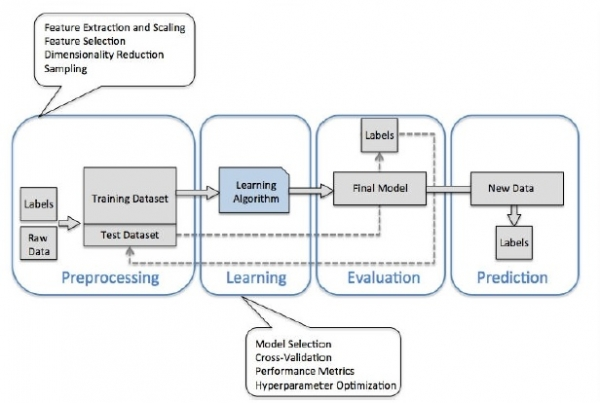# Learning Model Building in Scikit-learn: A Python Machine Learning Library

In this article, we will learn about the Learning Model Building in Scikit-learn: A Python Machine Learning Library.

It is a free machine learning library. It supports various algorithm like the random forest, vector machines & k-nearest neighbours with direct implementation with numpy and scipy.

## Importing the dataset

import pandas
Url = < specify your URL here>
data=pandas.rad_csv(url)

## Data exploration and cleaning

We can use the head method to specify/filter the records according to our needs.

data.head()
data.head(n=4) # restricting the record to be 4

We can also implement the last few records of the dataset

data.tail()
data.tail(n=4) # restricting the record to be 4

Now comes the stage of Data visualization

For this, we use the Seaborn module and matplotlib to visualize our data

import seaborn as s
import matplotlib.pyplot as plt
sns.set(style="whitegrid", color_codes=True)
# create a countplot
sns.countplot('Route To Market',data=sales_data,hue = 'Opportunity Result')

## Preprocessing the data

from sklearn import preprocessing
le = preprocessing.LabelEncoder()
#convert the columns into numeric values
encoded_value = le.fit_transform(list of column names)
print(encoded_value)

Finaly we reach the stage of Model building by training the data set.## Conclusion

In this article, we learnt about the model building in scikit-learn - a library available in Python.